# ISEE Middle Level Math : Data Analysis

## Example Questions

1 2 43 44 45 46 47 48 49 51 Next →

### Example Question #501 : Data Analysis

Use the following data set to answer the question: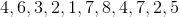Find the median.

Possible Answers:Correct answer:Explanation:

To find the median of a data set, we will first arrange the numbers within the set in ascending order.  Then, we will find the number in the middle of the set.

So, given the setwe will arrange the numbers in ascending order.  To do that, we will arrange them from smallest to largest.  So, we get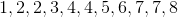Now, we will locate the number in the middle of the set.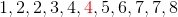We can see that it is 4.

Therefore, the median of the data set is 4.

### Example Question #502 : Data Analysis

Use the following data set to answer the question: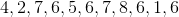Find the median.

Possible Answers:Correct answer:Explanation:

To find the median of a data set, we will first arrange the numbers within the set in ascending order, then we will locate the number in the middle of the set.

So, given the setwe will arrange them in ascending order.  To do that, we will arrange them from smallest to largest.  So, we get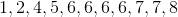Now, we will locate the number in the middle of the set.  So,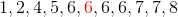We can see that it is 6.

Therefore, the median of the data set is 6.

### Example Question #503 : Data Analysis

A Math class took an exam.  Here are the scores of 11 students: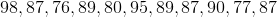Find the median score.

Possible Answers: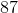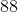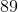Correct answer:Explanation:

To find the median score, we will first arrange the scores in ascending order.  Then, we will find the score in the middle of the set.

So, given the set of scoresWe will arrange the scores in ascending order.  To do that, we will arrange them from smallest to largest.  So, we get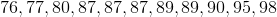Now, we will find the score in the middle of the set.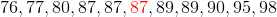Therefore, the median score is 87.

### Example Question #504 : Data Analysis

A class takes an exam.  Here are the scores of the 7 students who took the exam: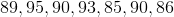Find the median score.

Possible Answers: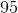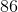Correct answer:Explanation:

To find the median score, we will first arrange the numbers in ascending order.  Then, we will find the number in the middle of the set.

So, given the setWe will arrange the scores in ascending order.  To do this, we will arrange them from smallest to largest.  So, we get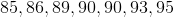Now, we will find the number in the middle of the set.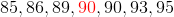We can see that it is 90.

Therefore, the median score is 90.

### Example Question #505 : Data Analysis

A Science class takes an exam.  Here are the results from 11 random students: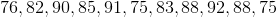Find the median score.

Possible Answers: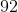Correct answer:Explanation:

To find the median score, we will arrange the scores in ascending order, then we will find the number in the middle.  So, in the data setNow, we will arrange them in ascending (from smallest to largest) order.  We get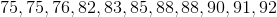And the number in the middle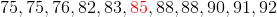Therefore, the median score is 85.

### Example Question #109 : How To Find Median

Identify the median of the numbers: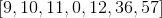Possible Answers:Correct answer:Explanation:

The median of an odd set of numbers is the central number of a chronologically ordered data set from least to greatest.

Rearrange the numbers from least to greatest.The central number is 11.

The answer is:### Example Question #506 : Data Analysis

Use the following data set to answer the question: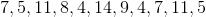Find the median.

Possible Answers:Correct answer:Explanation:

To find the median of a data set, we will first arrange the numbers in ascending order. Then, we will find the number in the middle of the set.

So, given the data setwe will arrange them in ascending order (from smallest to largest).  We get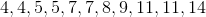Now, we will find the number in the middle of the set.  So,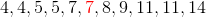We can see that it is 7.

Therefore, the median of the data set is 7.

1 2 43 44 45 46 47 48 49 51 Next →

### All ISEE Middle Level Math Resources